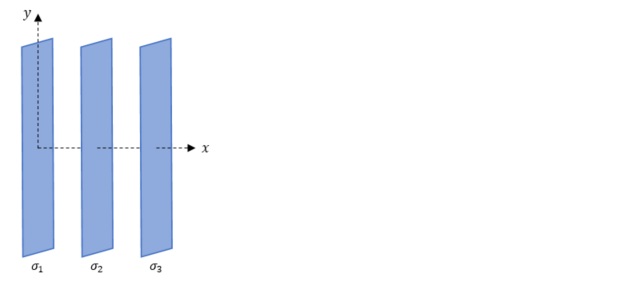# Problem: Consider the three infinite sheets of charge shown in the figure. The first sheet has chage density σ1 = +10μC/m2 and lies on the yz-plane. The second sheet has charge density of σ2 = +10μC/m2 and is parallel to the yz-plane at x = 0.10 m. The third sheet has charge density of σ3 = +10μC/m2 and is parallel to the yz-plane at x = 0.20 m. Determine the  electric field at the point P (0.05 m, 0).

82% (38 ratings)
###### Problem Details

Consider the three infinite sheets of charge shown in the figure. The first sheet has chage density σ1 = +10μC/m2 and lies on the yz-plane. The second sheet has charge density of σ2 = +10μC/mand is parallel to the yz-plane at x = 0.10 m. The third sheet has charge density of σ3 = +10μC/m2 and is parallel to the yz-plane at x = 0.20 m. Determine the  electric field at the point P (0.05 m, 0).What scientific concept do you need to know in order to solve this problem?

Our tutors have indicated that to solve this problem you will need to apply the Electric Fields in Capacitors concept. You can view video lessons to learn Electric Fields in Capacitors. Or if you need more Electric Fields in Capacitors practice, you can also practice Electric Fields in Capacitors practice problems.

What is the difficulty of this problem?

Our tutors rated the difficulty ofConsider the three infinite sheets of charge shown in the fi...as medium difficulty.

How long does this problem take to solve?

Our expert Physics tutor, Douglas took 8 minutes and 24 seconds to solve this problem. You can follow their steps in the video explanation above.

What professor is this problem relevant for?

Based on our data, we think this problem is relevant for Professor Hanna's class at PSU.